GRE Subject Test: Math : DeMorgan's Theorem

Example Questions

Example Question #141 : Other Topics

Using DeMorgan's law, which of the following is equivalent to the statement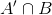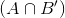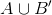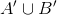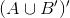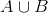Explanation:

The easiest way to remember DeMorgan's law is that you flip the symbol upside down (which changes union to intersection and vice versa), complement both sets (remembering that the complement of a complement is just that set), and either remove parentheses or add parentheses and place the complement symbol outside of itFlip the intersection symbol to a union, complement both sets, and add parentheses with the complement symbol outside of it.Example Question #1 : De Morgan's Theorem

Use DeMorgan's law to write a statement that is equivalent to the following statement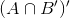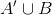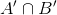Explanation:

The easiest way to remember DeMorgan's law is that you flip the symbol upside down (which changes union to intersection and vice versa), complement both sets (remembering that the complement of a complement is just that set), and either remove parentheses or add parentheses and place the complement symbol outside of it.Flip the symbol from an intersection to a union, complement both sets, and remove the parentheses.is equivalent to the original statement

Example Question #3 : De Morgan's Theorem

Using DeMorgan's law, is the statement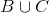equivalent to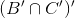? If not, choose the correct statement that is equivalent.

No, the equivalent statement is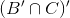No, the equivalent statement is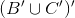Yes, the statements are equivalent.

No, the equivalent statement is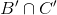No, the equivalent statement is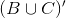Yes, the statements are equivalent.

Explanation:

The easiest way to remember DeMorgan's law is that you flip the symbol upside down (which changes union to intersection and vice versa), complement both sets (remembering that the complement of a complement is just that set), and either remove parentheses or add parentheses and place the complement symbol outside of it.Flip the union symbol to an intersection, complement both sets, add parentheses and a complement symbol outside the parentheses.Example Question #1 : De Morgan's Theorem

Using DeMorgan's law, is the statement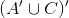equivalent to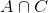? If not, choose the correct statement that is equivalent.

Yes, the statements are equivalent.

No, the equivalent statement is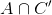No, the equivalent statement is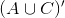No, the equivalent statement is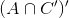No, the equivalent statement is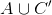No, the equivalent statement isExplanation:

The easiest way to remember DeMorgan's law is that you flip the symbol upside down (which changes union to intersection and vice versa), complement both sets (remembering that the complement of a complement is just that set), and either remove parentheses or add parentheses and place the complement symbol outside of it.Flip the union symbol to an intersection symbol, complement both sets, and remove the parenthesesExample Question #1 : De Morgan's Theorem

Using DeMorgan's law, which of the following is equivalent to the statement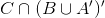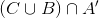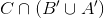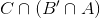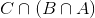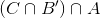Explanation:The first step is to realize that the C and intersection symbol in the original question are distractions and have nothing to do with applying DeMorgan's Law.

In the parentheses, flip the symbol from an intersection to a union and complement both sets.Normally, with only two sets, you should eliminate the parentheses that was there to show that the complement symbol applied to the entire parentheses. However, with three sets you still need to know the order in which to work out the problem so you should keep the parentheses around B and A.

Example Question #1 : De Morgan's Theorem

Using DeMorgan's law, is the statement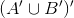equivalent to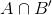? If not, choose the correct statement that is equivalent.

Yes, the statements are equivalent

No, the equivalent statement isNo, the equivalent statement is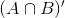No, the equivalent statement is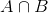No, the equivalent statement isNo, the equivalent statement isExplanation:

The easiest way to remember DeMorgan's law is that you flip the symbol upside down (which changes union to intersection and vice versa), complement both sets (remembering that the complement of a complement is just that set), and either remove parentheses or add parentheses and place the complement symbol outside of it.Flip the union symbol to an intersection symbol, complement both sets, and remove the parentheses.All GRE Subject Test: Math Resources